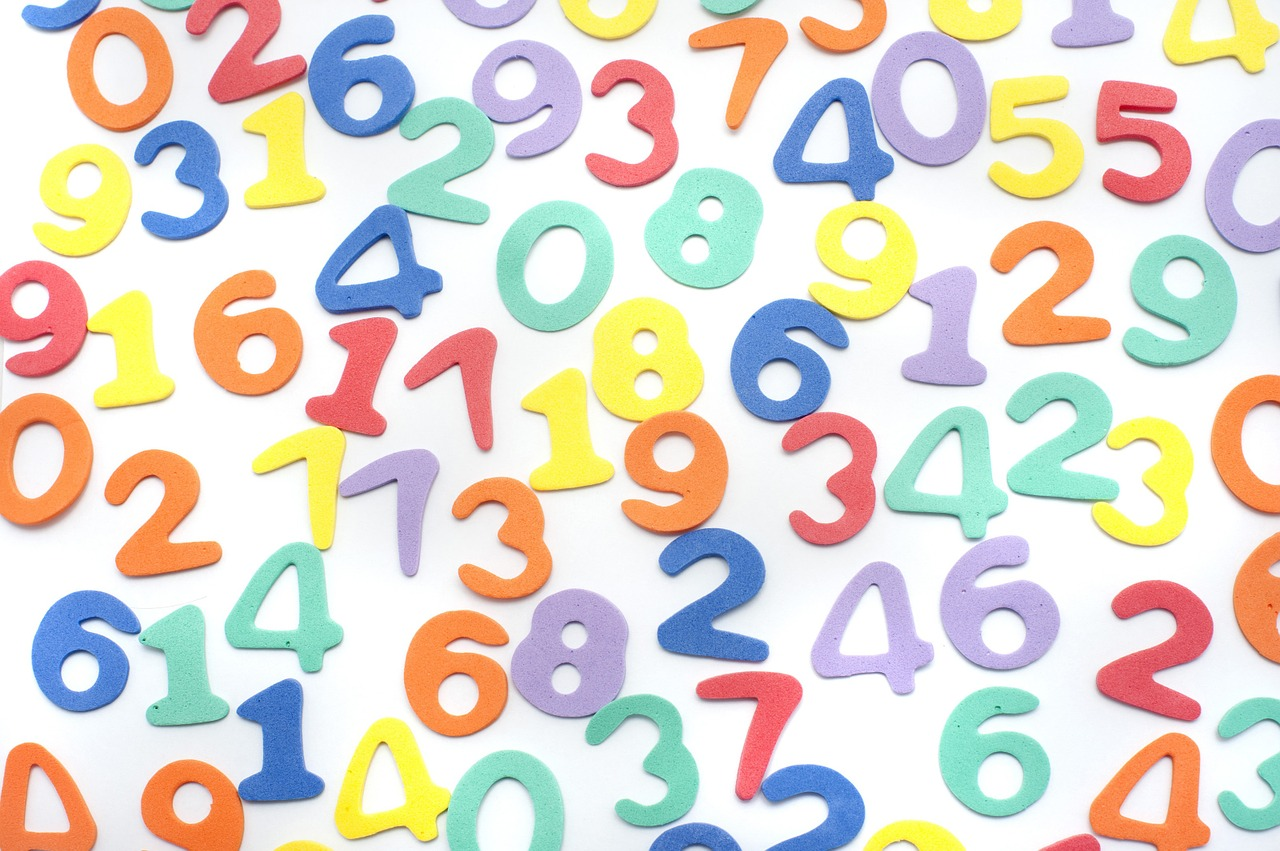## Math Program

Our in­-house developed math curriculum is designed for students to meet and exceed the BC Provincial Learning Outcomes within the subject. This math program covers math foundations from Kindergarten all the way through to excelling beyond the Grade 8 level. This course should be considered by those students who wish to move ahead of their current grade, or by those students who would benefit from extra math support. Our math tutoring classes allow students to work based on their own individual needs and pace. The students complete the math tutoring class in a setting of a maximum of 3 students with the support of an experienced math teacher, specialized at the level in which they teach.## Learning ObjectivesThis stage aims to build a solid foundation by teaching and consolidating the following areas of math utilizing the Singapore method:

• • Addition and subtraction and introduction to multiplication, division and simple fractions
• • Recognizing repeating and increasing/decreasing patterns
• • Recognizing and using mathematical symbols
• • Measuring and comparing length, mass, and capacity
• • Naming and comparing 2D shapes and 3D objects
• • Creating and interpreting a range of graphs
• • Financial literacy: Recognizing coin combinations to 100 cents, and understanding spending and savingThis stage continues to support students in accessing more complex mathematical concepts by teaching and consolidating the following:

• • Learn and utilize math vocabulary in word problems
• • The four operations
• • Variables and linear equations
• • Decimals, fractions, ratios and integers
• • Cartesian coordinates, coordinate planes, transformations and angles
• • Statistics, probability, algebra
• • Apply the Pythagorean theorem
• • Preservation of equality, different expressions, and equationsThis stage supports students in meeting core competencies in Pre-Calculus and workplace mathematics, ensuring a solid understanding of increasingly advanced concepts, including the following:

• • Perfect squares and cubes, surface area and volume
• • Square and cube roots
• • Percentages, proportional reasoning, fractions
• • Discrete and two-variable linear relations and expressions
• • Pythagorean theorem, trigonometry, multi-step equations
• • Foundational Pre-Calculus
• • Workplace mathematics
• • Statistics: central tendency, theoretical probability with two independent event
• • Financial literacy: budgets and proportional reasoning